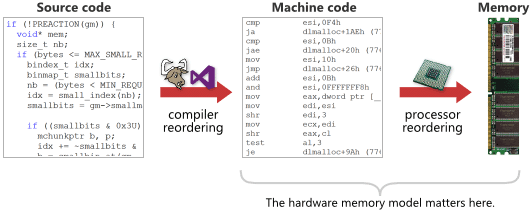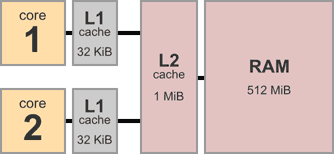#DAY 4
0

## Type of Memory Barrier

• Compiler Barrier
``````asm volatile("" ::: "memory");
``````
• x86 CPU Barrier
``````lfence (asm), void _mm_lfence(void)
sfence (asm), void _mm_sfence(void)
mfence (asm), void _mm_mfence(void)
``````
• ARMv7 CPU Barrier
``````dmb (asm)
dsb (asm)
isb (asm)
``````

`CPU Barrier` 會根據指令架構不同，有很大的差異

`Compiler Barrier``CPU Barrier` 的區別可以從下圖看出差別• L1
單顆 CPU 獨有，存取非常快速
• L2
多顆 CPU 共享
• 主記憶體
整個系統的記憶體，存取往往要消耗幾百個 Cycle

``````void thread1() {
asm("mov 1, [X]"   // store to X
"mov [Y], r1"  // load from Y
);
}

void thread2() {
asm("mov 1, [Y]"   // store to Y
"mov [X], r2"  // load from X
);
}
``````

• LoadLoad
如： `git pull`，一直去跟雲端要資料，在我們的例子中，是指去主記憶體抓取資料，保證數值是目前最新的，以下的範例是再回傳數值前作更新
``````if (IsPublished)                   // Load and check shared flag
{
LOADLOAD_FENCE();              // Prevent reordering of loads
return Value;                  // Load published value
}
``````
• StoreStore
如： `git push` 將資料存回雲端，在我們的例子中，是指把資料寫回主記憶體，對照到上一份程式碼，這表示要在 `IsPublished` 被設為 1 之前，寫回主記憶體
``````Value = x;                         // Publish some data
STORESTORE_FENCE();
IsPublished = 1;                   // Set shared flag to indicate availability of data
``````
• LoadStore
其實就是指 Data Dependency

假設先載入一個變數到暫存器，接著打算作寫入的動作，把資料寫回主記憶體

如果寫入的動作，有使用到前一個載入的變數，那麼就要確定變數已經確實載入，才能執行寫入的動作

但這邊指的 Barrier 都僅限於 Private Data，只是強調在個別 CPU 中的順序不能亂掉，而不是像 `StoreStore` 一定要先寫回主記憶體

• StoreLoad
將所有修改存回主記憶體，才繼續進行載入的動作，可以稱這樣的行為為「sequentially consistent」，所有指令都會按照撰寫順序進行

## Weak & Strong Memory Order• Really weak
完全不保證任何順序

• Weak with data dependency ordering
保證 `LoadLoad` 的順序

• Usually strong
保證 `LoadLoad` `StoreStore` `LoadStore` 的順序

• Sequentially consistent
保證全部的順序

## 範例

``````#define _GNU_SOURCE
#include <assert.h>
#include <pthread.h>
#include <sched.h>
#include <unistd.h>
#include <stdio.h>

static pthread_barrier_t barr, barr_end;
volatile int x, y, r1, r2;

static void* thread1(void *arg)
{
while(1) {
pthread_barrier_wait(&barr);
x = 1;    // store
//__asm__ __volatile__("mfence" ::: "memory");
r1 = y;   // load
pthread_barrier_wait(&barr_end);
}

return NULL;
}

static void* thread2(void *arg)
{
while (1) {
pthread_barrier_wait(&barr);
y = 1;   // store
//__asm__ __volatile__("mfence" ::: "memory");
r2 = x;  // load
pthread_barrier_wait(&barr_end);
}

return NULL;
}

int main()
{
pthread_barrier_init(&barr, NULL, 3);
pthread_barrier_init(&barr_end, NULL, 3);

pthread_t t1, t2;
pthread_create(&t1, NULL, thread1, NULL);
pthread_create(&t2, NULL, thread2, NULL);

//int num = sysconf(_SC_NPROCESSORS_CONF);

int cpu_1 = 0;
int cpu_2 = 1;
cpu_set_t cs;
CPU_ZERO(&cs);
CPU_SET(cpu_1, &cs);
pthread_setaffinity_np(t1, sizeof(cs), &cs);
CPU_ZERO(&cs);
CPU_SET(cpu_2, &cs);
pthread_setaffinity_np(t2, sizeof(cs), &cs);

// wait result
while(1) {
// init variable
x = y = r1 = r2 = 0;
pthread_barrier_wait(&barr);
pthread_barrier_wait(&barr_end);
printf("r1 = %d, r2 = %d\n", r1, r2);
//assert(!(r1 == 0 && r2 == 0));

}

pthread_barrier_destroy(&barr);
pthread_barrier_destroy(&barr_end);
return 0;
}
``````
• 透過 `CPU_SET` 以及 `pthread_setaffinity_np` 將 thread 限制在不同的 cpu 上執行
• 使用 `pthread_barrier` 進行等待，方便進行觀察

``````void thread1() {
.......
x = 1;
r1 = y;
.......
}

void thread2() {
.......
y = 1;
r2 = x;
.......
}
``````

## Others

Memory Barrier 這個鬼東西，我覺得很難理解，看了一堆心得我也搞不清楚有沒有解析錯誤，但如果要閱讀這系列文章，請務必要搞懂他

• Blocking Linked List
• Concurrent Linked List

BTW 我最原始想寫的 Lab 是開發一個閹割版的 `aria2`，可是這感覺會浪費一堆時間在搞各種連線的協 ......